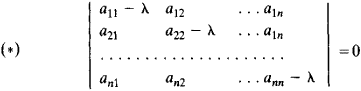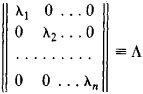# eigenvalue

(redirected from Eigenvalues)
Also found in: Dictionary, Thesaurus, Medical.

## Eigenvalue (quantum mechanics)

If an equation containing a variable parameter possesses nontrivial solutions only for certain special values of the parameter, these solutions are called eigenfunctions and the special values are called eigenvalues.

The eigenfunction-eigenvalue relation is of particular importance in quantum mechanics because of its prominence in the equations which relate the mathematical formalism of the theory with physical results. See Quantum mechanics

## Eigenvalue

(or characteristic value). An eigenvalue of a linear transformation or operator A is a number λ for which there exists a nonzero vector x such that Ax = λx; the vector x is called an eigenvector, or characteristic vector. Thus, the eigenvalues of a differential operator L(y) with given boundary conditions are numbers X for which the equation L(y) = λy has a nonzero solution that satisfies the boundary conditions. For example, if the operator L(y) has the form -y”, then numbers of the form λn = n2, where n is a natural number, are eigenvalues of the operator under the boundary conditions y (0) = y (π) = 0, since the functions yn = sin nx satisfy the equation - yn = n2y with the indicated boundary conditions. If, however, λ + n2 for any natural n, then only the function y(x) = 0 satisfies the equation –y” = λy under the same boundary conditions. Eigenvalues of linear operators are of importance in many problems in mathematics, mechanics, and physics—in problems, for example, in analytic geometry, algebra, the theory of vibrations, and quantum mechanics.

The eigenvalues of the matrix A = ║aik║, where i, k = 1, 2, . . . , n, are the eigenvalues of the linear transformation on an n-dimensional complex space that corresponds to A. The eigenvalues can also be defined as the roots of the equation det(A - λE) = 0, where E is the unit matrix—that is, the roots of the equationwhich is called the characteristic equation of the matrix. Since these numbers are the same for the similar matrices A and B’XAB where B is a nonsingular matrix, they characterize properties of the linear transformation that are independent of the choice of coordinate system. To each root λ, of equation (*) there corresponds a vector xi ≠ 0 (an eigenvector) such that Axi = λixi. If all the eigenvalues are distinct, then the set of eigenvectors may be chosen as the basis of the vector space. With respect to this basis, the linear transformation is described by the diagonal matrixEvery matrix A with distinct eigenvalues can be represented in the form C–1ʌC. If A is a Hermitian matrix, then its eigenvalues are real, the eigenvectors are orthogonal, and there exists a unitary matrix that can be chosen as C. The absolute value of every eigenvalue of a unitary matrix is equal to 1. The sum of the eigenvalues of a matrix is equal to the sum of its diagonal elements—that is, to the trace of the matrix. Knowledge of the eigenvalues of a matrix plays an important role in the investigation of the convergence of certain approximate methods of solving systems of linear equations.

## eigenvalue

[′ī·gən‚val·yü]
(mathematics)
The one of the scalars λ such that T (v) = λ v, where T is a linear operator on a vector space, and v is an eigenvector. Also known as characteristic number; characteristic root; characteristic value; latent root; proper value.

## eigenvalue

(mathematics)
The factor by which a linear transformation multiplies one of its eigenvectors.
References in periodicals archive ?
In this paper, we present a methodology to reduce the computational effort associated with repeated eigenvalue analyses in topography optimization of vibratory problems.
The results show that when the ANSYS Structural contact deflault selections are chosen - or the contact selections contain contact stiffness scale factors less than one, the complex eigenvalue solutions are uncorrelated and cannot be used to predict unstable eigenvalues (frequencies) or the potential for brake noise.
Primal constraints are then generated by using the eigenvectors of a few of the smallest eigenvalues of (2.
By Poincare's result, system (16) is formally integrable if and only if two eigenvalues of the matrix [MATHEMATICAL EXPRESSION NOT REPRODUCIBLE IN ASCII] satisfy condition (3).
The quadratic eigenvalue problem Q([lambda])x = 0 has exactly n eigenvalues [[lambda].
Since the algebraic multiplicity of nonzero eigenvalues of a compact operator is finite, each eigenvector of A - [lambda]I may have only a finite number of linear independent associated vectors.
In brief, the PA method consists of: (a) simulating a large number of normal random samples that parallel the observed data in terms of sample size and number of variables; (b) computing correlation matrices from the "uncorrelated-data" generated in a) and extracting the eigenvalues from the correlation matrices in b); and (c) comparing the observed eigenvalues (in your data) with the "null eigenvalues" obtained in c).
Inequalities for the Steklov Eigenvalues, arXiv:1006.
The eigenvalues of a diagonalizable matrix A whose eigenvectors span j are said to be main.

Site: Follow: Share:
Open / Close